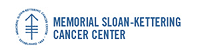## Memorial Sloan-Kettering Cancer Center, Dept. of Epidemiology & Biostatistics Working Paper Series

#### Title

Smoothed Rank Regression with Censored Data

#### Abstract

A weighted rank estimating function is proposed to estimate the regression parameter vector in an accelerated failure time model with right censored data. In general, rank estimating functions are discontinuous in the regression parameter, creating difficulties in determining the asymptotic distribution of the estimator. A local distribution function is used to create a rank based estimating function that is continuous and monotone in the regression parameter vector. A weight is included in the estimating function to produce a bounded influence estimate. The asymptotic distribution of the regression estimator is developed and simulations are performed to examine its finite sample properties. A lung cancer data set is used to illustrate the methodology.

#### Disciplines

Survival Analysis

COinS